Are you more of a visual learner? Check out our online video lectures and start your physics course now for free!Image: “Kugelstoßpendel” by Bastian Greshake. License: CC BY-SA 2.0

## Oscillation

Oscillations are processes in which a physical quantity changes periodically for a period of time. These include movements that occur periodically around an idle position.

The most significant vibration form is the harmonic vibration; this periodic change of a physical quantity is sinusoidal. All other vibration modes are not harmonious.

## Basic Concepts of Oscillations and Waves

• Complete back-and-forth movement of the oscillating body is called a period.
• The amplitude is the extent of a wave measured from the highest peak down to the zero position.
• The period duration T is the time needed for an oscillating body to move back and forth, i.e. to fulfill one period. It is often referred to as the period.
• Frequency is the quotient of the number of periods and the time required for it.
• Elongation is defined as the current distance from the zero position.
• Circular frequency is the angular velocity of a circular movement whose projection on a straight line results in a harmonic oscillation.
• The phase is specified by the two oscillation parameters—elongation and time—and is indicative of the current state of oscillation.
• The damped oscillation is an oscillation in which the amplitude of the oscillating quantity decreases with time because of frictional forces.
• Undamped oscillations have constant amplitudes. It is necessary for the energy supplied to the vibrating system to be maintained. In the case of constant small energy losses, a sustained undamped oscillation, in reality, is only approximately possible. In order to create a real undamped oscillation, the energy losses that occur must be compensated for by a regular energy supply.

## Undamped Harmonic Oscillation

Harmonic oscillations are also referred to as sine oscillations. The reason for this is that the harmonic oscillation elongation is a sinusoidal function of time.

The harmonic oscillation is an irregular accelerated motion, and its acceleration is a function of time. Find out more in the articles ‘Mechanics Part I’ and ‘Mechanics Part II.’

At any given time during harmonic oscillation, a force acts in the direction of acceleration that wants to bring the oscillating body back to its center position. This force is known as the restoring force. This restoring force is proportional to the elongation.

The base equation of dynamics for mechanical oscillation is as follows:

restoring force = mass × acceleration of gravity

Fr = m × a

From this principle, the equation of the undamped harmonic oscillation can be derived:

ӱ + y × ω2 = 0

where y denotes elongation and ω angular frequency.

## Forced Oscillations

Whenever an oscillatory system is displaced and released from its zero position, it will begin to oscillate. If no other external forces are applied, it is a free oscillation. The frequency of the free oscillation is called ‘natural frequency,’ or ‘eigenfrequency.’

When an external periodic driving force is imposed on the oscillating system to keep it going, the oscillation is known as forced oscillationThree forces are acting on the oscillating system, as follows:

1. Conservation force
2. Damping force
3. Excitation force

## Resonance

Resonance occurs when the excitation frequency matches the natural frequency of the oscillation. When the damping is small, the amplitude decreases greatly. In everyday life—and in technology—resonance plays a vital role.

Because most mechanical structures are subject to an oscillating force, they can be stimulated by external periodic forces. In the case of resonance, this would result in an increase of amplitude followed by the destruction of the structure.

To prevent such a ‘resonance catastrophe,’ one has to avoid any periodic forces or maintain large margins between the natural frequency and the excitation frequency, provide damping options, and allow a resonance frequency for only a short time—a time that is less than the buildup time. For rotary movements, the resonance frequency is referred to as a critical rotational speed.

## Superposition of Oscillations

Each oscillating system can execute several oscillations simultaneously. The individual oscillations overlap and form a resultant oscillation. In conclusion, if the oscillating bodies execute several oscillations, they then superpose themselves without interfering with each other.

A distinction is made between oscillations that possess the same oscillating directions and oscillations whose directions of oscillation are perpendicular to each other. If two harmonic vibrations with the same direction and the same frequency are superposed, what results is a harmonic oscillation of the same frequency, whose amplitude depends on the individual amplitudes. The phases of the initial oscillations also affect the amplitude of the resulting oscillations.

When two oscillations in the same direction, but not the same frequency, are superposed, the result is a nonharmonic oscillation.

## Waves

The oscillation process in an extended medium is called an oscillating wave. The extended medium consists of a vast amount of oscillating particles, all of which are interconnected. If one of these particles receives an oscillation impulse, it then becomes the center of a propagating wave movement.

A wave is a spatial and temporary periodic process in which energy—but no matter—is transported. The direction of energy propagation is called the wave ray. Perpendicular to the wave ray runs the wavefront. The wavefront is the geometric location of all the particles that belong to the same phase.

The distance between two successive wavefronts is called the wavelength. The distance between two neighboring particles of the same oscillation phase is the wave propagation, which is independent of location and time. The wave propagation can be analyzed according to Huygens’ principle of wavelets. In this principle, every point of a medium covered by a wave motion can be regarded as the starting point of a new wave—a so-called wavelet. Each wavefront conforms to the envelope of wavelets. Huygens’ principle applies to all types of waves, even electromagnetic waves.

### Wave types

• Longitudinal waves: The particles oscillate back and forth in the direction of propagation. If the particles oscillate in the direction of travel of the wave, an overpressure (compression) occurs. If they oscillate opposite to the direction of wave travel, a vacuum (rarefaction) occurs. Compressions and rarefactions alternate.
• Transverse waves: The particles oscillate perpendicular to the direction of travel of the wave. Transverse waves have alternating troughs and crests.
• Plane waves are one-dimensional waves. They travel in only one direction.
• Surface waves are two-dimensional waves. They propagate in an expanding surface.
• Sky waves are three-dimensional waves. They propagate in the form of an expanding sphere.

When surface waves have a punctiform excitation center, the wavefronts are circles. When sky waves have a punctiform excitation center, the wavefronts are spherical shells.

The velocity of wave propagation c is equal to the product of the frequency f with which each particle of the wave oscillates and its wavelength λ:

c = f λ

The velocity of wave propagation is given by the following equations:

Elastic transverse wave in solids: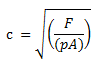Elastic longitudinal wave in solids: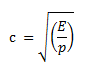Longitudinal wave in liquids: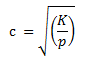where c denotes propagation velocity, F tension force, A cross-sectional area, ρ density of the medium, E modulus of elasticity, and K bulk modulus.

If two waves superpose and coincide in amplitude, frequency, and wavelength but run in opposite directions, a so-called standing wave occurs. In a standing wave, the spatial image does not travel any further. Locations at which the amplitude is maximum (antinodes) and locations at which amplitude is minimum or equal to 0 (nodes) remain in a constant position.

Standing waves can occur with the reflection on a thinner or a denser medium. A standing wave occurs most frequently when a plane wave superposes with itself after a reflection.

## Reflection

When a wave strikes the boundary of its medium onto another medium, it is entirely or partially reflected. This process is called ‘reflection.’ The law of reflection is as follows:

angle of incidence = angle of reflection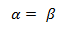A wave ray is refracted during the transition between two media, and the propagation direction and the propagation speed change. For the propagation velocities, the law of refraction applies:

##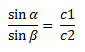Diffraction

Further change in the propagation velocity of the wave can be found at the edge of an obstacle, such as a slit. The obstacle does not cast sharp shadows. Huygens’ principle can explain the phenomenon of diffraction, as shown below.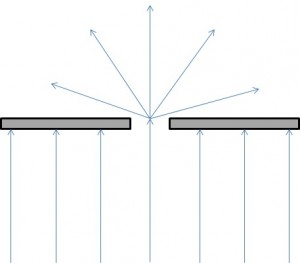The energy of the wave-particle diffracted through the opening slit of the wall is distributed in individual directions after diffraction so that the energy parts decrease with increasing diffraction angle. The diffraction angle is the angle between the original wave direction and the new wave directions.

## Electromagnetic Spectrum

The electromagnetic wave is caused by the oscillation of the coupled electric and magnetic fields. They are dependent on the frequency.

Electromagnetic waves can propagate both in free space and in a vacuum. They do not need a carrier medium. In a vacuum, these waves propagate at the speed of light. The speed of light in a vacuum is, according to current measurements, c = (299,792.5 ± 0.9) km/s.

## Infrared Light

The white light of an arc lamp or light bulb provides invisible radiation that can be found in the visible spectrum next to red. It is referred to as ‘infrared radiation’ and results in infrared light.

## Ultraviolet Light

The ultraviolet color range lies outside the violet range of the visible color spectrum. Irritation and tanning of the skin by the sun are due to the ultraviolet light component. Mercury vapor lamps that are used as artificial tanning lamps also emit ultraviolet light.

The different impressions of color are projected to our eye by different frequencies of the visible spectrum:

Apart from the nuclear components—protons, neutrons, and electrons—today, more than 200 other elementary particles are known. Many of them are the result of the interactions between the terrestrial atmosphere and cosmic radiation or the product of nuclear disintegrations supported by particle accelerators. The elementary particles are classified into the following groups:

• Leptons: light particles
• Mesons: medium-weight particles
• Baryons: heavy particles

Most elementary particles exist with an antipole electric charge and a reversed magnetic momentum; these are so-called antiparticles. If a particle meets with its antiparticle, the result is annihilation. The energy of annihilation is released as gamma radiation.

## Sound Waves

Sound waves are mechanical longitudinal waves. They have their origin in the sound source (a vibrating body) and propagate in solids, liquids, and gases in the form of compression waves.

Example: The human ear can hear frequencies from 16 Hz to 20,000 Hz. Frequencies lower than 16 Hz are called infrasound. Frequencies higher than 20,000 Hz are called ultrasound.

In acoustics, distinctions are made among the following types of sounds:

• Tone: The pure tone is graphically displayed as a sinusoidal wave. The frequency specifies the pitch of the tone. The ratio between two tones is called the ‘interval.’ In musical intervals, the lowest note is called the ‘root’the fundamental tone. The higher notes are the octave, the perfect fifth, the perfect fourth, etc. The musical interval of two tones, which can be detected by the sense of hearing, is defined by the quotient of their frequencies.
• Sound: Several sinusoidal oscillations superpose to a nonsinusoidal vibration. The pitch is determined by the root; the other sounds convey the timbre.
• Noise: A variety of sounds with rapidly changing frequencies and strength.
• Bang: A sudden loud noise that lasts for a very brief time.

## Sound

Everything perceived with the human ear is called sound. We distinguish between tones and noises. The way we perceive a sound event depends on its volume, pitch, and timbre. Sound comes from a sound generator—which is an oscillatory body.

In order for sound to reach our ears, it must be transmitted by a sound carrier. The propagation of sound requires a solid, liquid, or gaseous body as a sound carrier. Sound cannot be transmitted in a vacuum. The sound source generates progressive longitudinal waves in the sound carrier. The perception of sound occurs when these longitudinal waves reach our ears (sound receivers).

## Speed of Sound

The speed of sound specifies how fast the sound propagates in a particular sound carrier. It is independent of frequency. In the air, for example, the speed of sound is 340 m/s and in water 1440 m/s.

When sound waves hit another medium, they are reflected at the transition boundary.

Example: In a sonographic measurement, frequencies of a specific size transmitted by a sound source are reflected at an interface (e.g., tissue or blood components in the Doppler method) and picked up with a measuring probe in a changed frequency. The distance covered can be calculated from the required time, given a known sound propagation speed (or known initial frequencies).
Learn. Apply. Retain.
Your path to achieve medical excellence.
Study for medical school and boards with Lecturio.